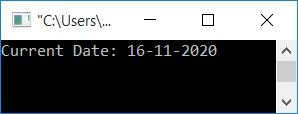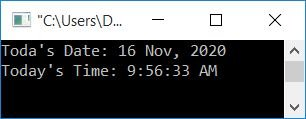# C program to print date and time

In this article, you will learn and get code about the printing of date and time in the following ways:

## Print the date and time in the default format

Let's create a program that prints the current date and time in its default format.

```#include<stdio.h>
#include<conio.h>
#include<time.h>
int main()
{
time_t tm;
time(&tm);
printf("Current Date/Time = %s", ctime(&tm));
getch();
return 0;
}```

This program was built and runs in the the Code::Blocks IDE. Here is its sample run:The time_t is not a primitive data type; rather, it is a data type from the C ISO library defined to store a system's time value. This system's time values are returned from the standard library function named time().

The function ctime() returns a string that represents the local time based on the tm (argument) timer. The ctime() function is defined in the time.h header file.

## Print the date in DD-MM-YYYY format

Now let's format the current date in DD-MM-YYYY form, where DD represents the day's date (1-31), MM represents the month (1-12), and YYYY represents the year.

```#include<stdio.h>
#include<conio.h>
#include<time.h>
int main()
{
time_t t;
t = time(NULL);
struct tm tm = *localtime(&t);
printf("Current Date: %d-%d-%d", tm.tm_mday, tm.tm_mon+1, tm.tm_year+1900);
getch();
return 0;
}```

Here is its sample output:The function localtime() returns the local time of the system. It is defined in the time.h header file. It accepts a parameter that represents the pointer to the time_t object. and returns a pointer to a "struct tm" object. Now, using the structure, the object tm can access the data members, say tm_mday, tm_mon, and tm_year, respectively. Print its value as output, which will be the date, month, and year.

Note: The tm_year field is relative to 1900 on all POSIX-compliant platforms. Therefore, we have added 1900 to it. If 1900 was not added to it, then in place of 2020 as the current year, it would print 120 as the current year, which means it has been 120 years since 1900.

It should be noted that the tm_mon is displayed starting from 1 rather than 0.Because if 1 is not added, then for January it will show the 0th month (the starting or very first month of every year).

## Print the time in the HH:MM:SS format

Let's create the same program as the previous one with a few changes to print time in HH:MM:SS format in place of date. Here HH represents hours, MM represents minutes, and SS represents seconds.

```#include<stdio.h>
#include<conio.h>
#include<time.h>
int main()
{
time_t t;
t = time(NULL);
struct tm tm;
tm = *localtime(&t);
printf("Current Time: %d:%d:%d", tm.tm_hour, tm.tm_min, tm.tm_sec);
getch();
return 0;
}```

Here is its sample output:## Print the date and time, along with the month name and AM/PM

This program uses a switch case to match the month number and print its name. For example, if the month number is 1, then print it as Jan; if it is 2, then print it as Feb; and so on. For time, we have used an if-else statement to match whether the current hour value is greater than 12 or not. If it is greater than 12, then print PM; otherwise, print AM.

```#include<stdio.h>
#include<conio.h>
#include<time.h>
int main()
{
time_t t;
t = time(NULL);
struct tm tm = *localtime(&t);
int m;
printf("Today's Date: %d ", tm.tm_mday);
m = tm.tm_mon+1;
switch(m)
{
case 1:
printf("Jan, ");
break;
case 2:
printf("Feb, ");
break;
case 3:
printf("Mar, ");
break;
case 4:
printf("Apr, ");
break;
case 5:
printf("May, ");
break;
case 6:
printf("June, ");
break;
case 7:
printf("July, ");
break;
case 8:
printf("Aug, ");
break;
case 9:
printf("Sep, ");
break;
case 10:
printf("Oct, ");
break;
case 11:
printf("Nov, ");
break;
case 12:
printf("Dec, ");
break;
}
printf("%d", tm.tm_year+1900);
printf("\nToday's Time: ");
if(tm.tm_hour>=12)
{
if(tm.tm_hour==12)
printf("12");
else
printf("%d", tm.tm_hour-12);
printf(":%d:%d PM", tm.tm_min, tm.tm_sec);
}
else
printf("%d:%d:%d AM", tm.tm_hour, tm.tm_min, tm.tm_sec);
getch();
return 0;
}```

Here is the sample output produced by the above program:#### The same program in different languages

C Quiz

« Previous Program Next Program »